# Einops tutorial, part 1: basics¶

## Welcome to einops-land!¶

We don't write

y = x.transpose(0, 2, 3, 1)


We write comprehensible code

y = rearrange(x, 'b c h w -> b h w c')


einops supports widely used tensor packages (such as numpy, pytorch, chainer, gluon, tensorflow), and extends them.

## What's in this tutorial?¶

• fundamentals: reordering, composition and decomposition of axes
• operations: rearrange, reduce, repeat
• how much you can do with a single operation!

## Preparations¶

In :
# Examples are given for numpy. This code also setups ipython/jupyter
# so that numpy arrays in the output are displayed as images
import numpy
from utils import display_np_arrays_as_images
display_np_arrays_as_images()


## Load a batch of images to play with¶

In :
ims = numpy.load('./resources/test_images.npy', allow_pickle=False)
# There are 6 images of shape 96x96 with 3 color channels packed into tensor
print(ims.shape, ims.dtype)

(6, 96, 96, 3) float64

In :
# display the first image (whole 4d tensor can't be rendered)
ims

Out:In :
# second image in a batch
ims

Out:In :
# we'll use three operations
from einops import rearrange, reduce, repeat

In :
# rearrange, as its name suggests, rearranges elements
# below we swapped height and width.
# In other words, transposed first two axes (dimensions)
rearrange(ims, 'h w c -> w h c')

Out:## Composition of axes¶

transposition is very common and useful, but let's move to other capabilities provided by einops

In :
# einops allows seamlessly composing batch and height to a new height dimension
# We just rendered all images by collapsing to 3d tensor!
rearrange(ims, 'b h w c -> (b h) w c')

Out:In :
# or compose a new dimension of batch and width
rearrange(ims, 'b h w c -> h (b w) c')

Out:In :
# resulting dimensions are computed very simply
# length of newly composed axis is a product of components
# [6, 96, 96, 3] -> [96, (6 * 96), 3]
rearrange(ims, 'b h w c -> h (b w) c').shape

Out:
(96, 576, 3)
In :
# we can compose more than two axes.
# let's flatten 4d array into 1d, resulting array has as many elements as the original
rearrange(ims, 'b h w c -> (b h w c)').shape

Out:
(165888,)

## Decomposition of axis¶

In :
# decomposition is the inverse process - represent an axis as a combination of new axes
# several decompositions possible, so b1=2 is to decompose 6 to b1=2 and b2=3
rearrange(ims, '(b1 b2) h w c -> b1 b2 h w c ', b1=2).shape

Out:
(2, 3, 96, 96, 3)
In :
# finally, combine composition and decomposition:
rearrange(ims, '(b1 b2) h w c -> (b1 h) (b2 w) c ', b1=2)

Out: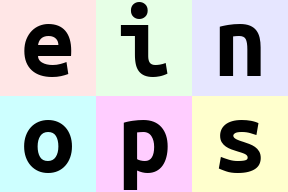In :
# slightly different composition: b1 is merged with width, b2 with height
# ... so letters are ordered by w then by h
rearrange(ims, '(b1 b2) h w c -> (b2 h) (b1 w) c ', b1=2)

Out:In :
# move part of width dimension to height.
# we should call this width-to-height as image width shrinked by 2 and height doubled.
# but all pixels are the same!
# Can you write reverse operation (height-to-width)?
rearrange(ims, 'b h (w w2) c -> (h w2) (b w) c', w2=2)

Out:## Order of axes matters¶

In :
# compare with the next example
rearrange(ims, 'b h w c -> h (b w) c')

Out:In :
# order of axes in composition is different
# rule is just as for digits in the number: leftmost digit is the most significant,
# while neighboring numbers differ in the rightmost axis.

# you can also think of this as lexicographic sort
rearrange(ims, 'b h w c -> h (w b) c')

Out:In :
# what if b1 and b2 are reordered before composing to width?
rearrange(ims, '(b1 b2) h w c -> h (b1 b2 w) c ', b1=2) # produces 'einops'
rearrange(ims, '(b1 b2) h w c -> h (b2 b1 w) c ', b1=2) # produces 'eoipns'

Out:## Meet einops.reduce¶

In einops-land you don't need to guess what happened

x.mean(-1)


Because you write what the operation does

reduce(x, 'b h w c -> b h w', 'mean')


if axis is not present in the output — you guessed it — axis was reduced.

In :
# average over batch
reduce(ims, 'b h w c -> h w c', 'mean')

Out:In :
# the previous is identical to familiar:
ims.mean(axis=0)
# but is so much more readable

Out:In :
# Example of reducing of several axes
# besides mean, there are also min, max, sum, prod
reduce(ims, 'b h w c -> h w', 'min')

Out:In :
# this is mean-pooling with 2x2 kernel
# image is split into 2x2 patches, each patch is averaged
reduce(ims, 'b (h h2) (w w2) c -> h (b w) c', 'mean', h2=2, w2=2)

Out: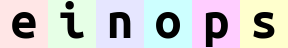In :
# max-pooling is similar
# result is not as smmoth as for mean-pooling
reduce(ims, 'b (h h2) (w w2) c -> h (b w) c', 'max', h2=2, w2=2)

Out: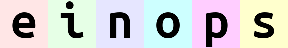In :
# yet another example. Can you compute result shape?
reduce(ims, '(b1 b2) h w c -> (b2 h) (b1 w)', 'mean', b1=2)

Out:## Stack and concatenate¶

In :
# rearrange can also take care of lists of arrays with the same shape
x = list(ims)
print(type(x), 'with', len(x), 'tensors of shape', x.shape)
# that's how we can stack inputs
# "list axis" becomes first ("b" in this case), and we left it there
rearrange(x, 'b h w c -> b h w c').shape

<class 'list'> with 6 tensors of shape (96, 96, 3)

Out:
(6, 96, 96, 3)
In :
# but new axis can appear in the other place:
rearrange(x, 'b h w c -> h w c b').shape

Out:
(96, 96, 3, 6)
In :
# that's equivalent to numpy stacking
numpy.array_equal(rearrange(x, 'b h w c -> h w c b'), numpy.stack(x, axis=3))

Out:
True
In :
# ... or we can concatenate
rearrange(x, 'b h w c -> h (b w) c').shape  # numpy.stack(x, axis=3))

Out:
(96, 576, 3)
In :
# which is behavior of concatenation
numpy.array_equal(rearrange(x, 'b h w c -> h (b w) c'), numpy.concatenate(x, axis=1))

Out:
True

## Addition or removal of axes¶

You can write 1 to create new axis of length 1. Similarly you can remove such axis.

There is also a synonym () that you can use. That's a composition of zero axes and it also has a unit length.

In :
x = rearrange(ims, 'b h w c -> b 1 h w 1 c') # functionality of numpy.expand_dims
print(x.shape)
print(rearrange(x, 'b 1 h w 1 c -> b h w c').shape) # functionality of numpy.squeeze

(6, 1, 96, 96, 1, 3)
(6, 96, 96, 3)


## Reduce ⇆ repeat¶

reduce and repeat are like opposite of each other: first one reduces amount of elements, second one increases

In :
# compute max in each image individually, then show a difference
x = reduce(ims, 'b h w c -> b () () c', 'max') - ims
rearrange(x, 'b h w c -> h (b w) c')

Out:## Fancy examples in random order¶

(a.k.a. mad designer gallery)

In :
# interweaving pixels of different pictures
# all letters are observable
rearrange(ims, '(b1 b2) h w c -> (h b1) (w b2) c ', b1=2)

Out:In :
# interweaving along vertical for couples of images
rearrange(ims, '(b1 b2) h w c -> (h b1) (b2 w) c', b1=2)

Out: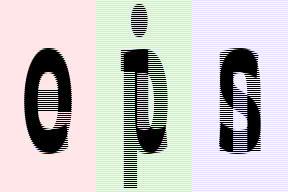In :
# interweaving lines for couples of images
# exercise: achieve the same result without einops in your favourite framework
reduce(ims, '(b1 b2) h w c -> h (b2 w) c', 'max', b1=2)

Out:In :
# color can be also composed into dimension
# ... while image is downsampled
reduce(ims, 'b (h 2) (w 2) c -> (c h) (b w)', 'mean')

Out: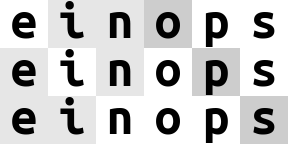In :
# disproportionate resize
reduce(ims, 'b (h 4) (w 3) c -> (h) (b w)', 'mean')

Out: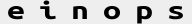In :
# spilt each image in two halves, compute mean of the two
reduce(ims, 'b (h1 h2) w c -> h2 (b w)', 'mean', h1=2)

Out: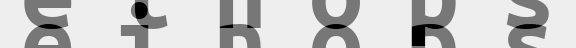In :
# split in small patches and transpose each patch
rearrange(ims, 'b (h1 h2) (w1 w2) c -> (h1 w2) (b w1 h2) c', h2=8, w2=8)

Out:In :
# stop me someone!
rearrange(ims, 'b (h1 h2 h3) (w1 w2 w3) c -> (h1 w2 h3) (b w1 h2 w3) c', h2=2, w2=2, w3=2, h3=2)

Out:In :
rearrange(ims, '(b1 b2) (h1 h2) (w1 w2) c -> (h1 b1 h2) (w1 b2 w2) c', h1=3, w1=3, b2=3)

Out:In :
# patterns can be arbitrarily complicated
reduce(ims, '(b1 b2) (h1 h2 h3) (w1 w2 w3) c -> (h1 w1 h3) (b1 w2 h2 w3 b2) c', 'mean',
h2=2, w1=2, w3=2, h3=2, b2=2)

Out: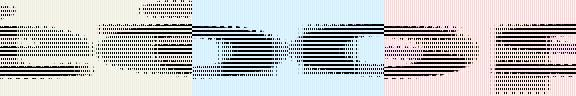In :
# subtract background in each image individually and normalize
# pay attention to () - this is composition of 0 axis, a dummy axis with 1 element.
im2 = reduce(ims, 'b h w c -> b () () c', 'max') - ims
im2 /= reduce(im2, 'b h w c -> b () () c', 'max')
rearrange(im2, 'b h w c -> h (b w) c')

Out:In :
# pixelate: first downscale by averaging, then upscale back using the same pattern
averaged = reduce(ims, 'b (h h2) (w w2) c -> b h w c', 'mean', h2=6, w2=8)
repeat(averaged, 'b h w c -> (h h2) (b w w2) c', h2=6, w2=8)

Out:In :
rearrange(ims, 'b h w c -> w (b h) c')

Out:In :
# let's bring color dimension as part of horizontal axis
# at the same time horizonal axis is downsampled by 2x
reduce(ims, 'b (h h2) (w w2) c -> (h w2) (b w c)', 'mean', h2=3, w2=3)

Out:## Ok, numpy is fun, but how do I use einops with some other framework?¶

If that's what you've done with ims being numpy array:

rearrange(ims, 'b h w c -> w (b h) c')


That's how you adapt the code for other frameworks:

# pytorch:
rearrange(ims, 'b h w c -> w (b h) c')
# tensorflow:
rearrange(ims, 'b h w c -> w (b h) c')
# chainer:
rearrange(ims, 'b h w c -> w (b h) c')
# gluon:
rearrange(ims, 'b h w c -> w (b h) c')
# cupy:
rearrange(ims, 'b h w c -> w (b h) c')
# jax:
rearrange(ims, 'b h w c -> w (b h) c')

...well, you got the idea.


Einops allows backpropagation as if all operations were native to framework. Operations do not change when moving to another framework

# Summary¶

• rearrange doesn't change number of elements and covers different numpy functions (like transpose, reshape, stack, concatenate, squeeze and expand_dims)
• reduce combines same reordering syntax with reductions (mean, min, max, sum, prod, and any others)
• repeat additionally covers repeating and tiling
• composition and decomposition of axes are a corner stone, they can and should be used together